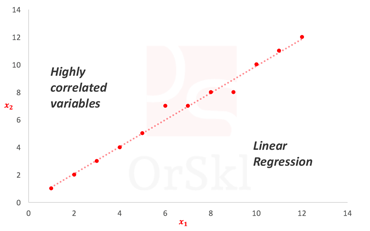hello dummmy textLinear regression is one of the basic and widely used machine learning algorithms in the area of data science and analytics for predictions. Data scientists will deal with huge dimensions of data and it will be quite challenging to build simplest linear model possible. In this process of building the model there are possibilities of two or more variables (also called predictors) highly correlated to each other. Let us try to understand with very simple data having highly correlated predictors and how does it affect the regression or linear equation mathematically. For the same data set, we will try to understand the regression behavior in Python with and without having correlated variable(s).

Intuitive understanding of mathematical concepts will help you implement the same in the real world problems. So, why you should really worry of correlated variables in the data set? Let us quickly start off with the data set below.

If you are asked to write a linear equation for the above grid with the basic math skills of trial and errors, we can come up with following combinations and in fact there would be many more.

Of these three possibilities, (3) is better to choose as you can compute the value of  with only one variable . But it also means that , , are highly correlated variables. Let us compute and validate the correlations between each of these variables.

Clearly says that all three columns are positively & highly correlated and hence it is viable to use only one variable instead of all three as the information that exists in  or   or   is good enough to compute .

Challenge is, any machine learning models cannot automatically identify these highly correlated variables and pick only one of these. This is where data scientists are needed to deal with such situations.

Let us build linear regression model in python and read the outcome.

``````import matplotlib.pyplot as plt

import numpy as np

import pandas as pd

from sklearn import datasets, linear_model, metrics

from sklearn.model_selection import train_test_split

import statsmodels.api as sm

from statsmodels.stats.outliers_influence import variance_inflation_factor

x=pd.DataFrame({‘x1′:[1,2,3,4,5,6,7],’x2′:[2,3,4,5,6,7,8],’x3’:[2,3,4,5,6,7,8]})

y=pd.DataFrame({‘y’:[5,8,11,14,17,20,23]})

reg = linear_model.LinearRegression()

reg_output=reg.fit(x,y)

print(‘Coefficients: \n’, reg_output.coef_)

(‘Coefficients: \n’, array([[1., 1., 1.]]))``````

So the linear regression model has build  =  +   + , (1) from the above list, as system by itself cannot handle multicollinearity in the variables. There are methods for data scientists to identify highly correlated variables. One of the most commonly used methods is Variation Inflation Factor (VIF). A VIF value of > 10 means that the variable is more than 90% correlation with other variables in the dataset and we can choose to remove these variables and re-run the model.

``````vif = pd.DataFrame()

vif[“VIF Factor”] = [variance_inflation_factor(x.values, i) for i in range(x.shape)]
vif[“features”] = x.columns

vif

VIF Factor features

0    145       x1

1    inf       x2

2    inf       x3``````

inf is infinity as the correlation is 1 exactly. So we remove both  and variables from the data and rebuild the model.

``````X=sm.add_constant(x[“x1”])

reg_output_x1=sm.OLS(y,X).fit()

reg_output_x1.summary()

coef      std err   t         P>|t| [0.025    0.975]
const     2.0000    7.81e-15  2.56e+14  0.000 2.000      2.000

x1        3.0000    1.75e-15  1.72e+15  0.000 3.000 3.000``````

So the model with only  has built the equation as ,  =3  + 2 (3) one in the list above.

Conclusion:

By treating multicollinearity in the variables, machine learns to build possibly simple predictive model with minimum variables we pass.

For larger data set removing highly collinear features will help to build models relatively faster.

Model would become sensitive with highly collinear variables, which means that with small variations in the data, model would return large residual errors in the prediction.

Do you wish to watch video for the same? Here you go.